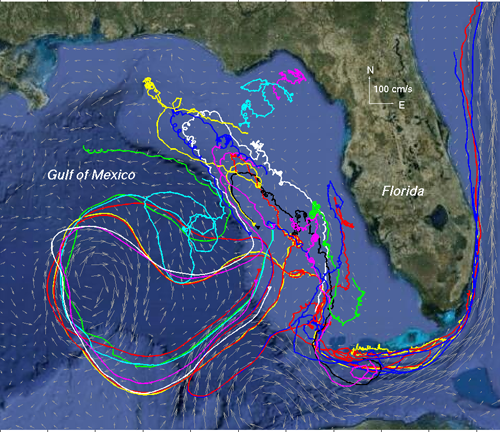## Evaluation of trajectory modeling in different dynamic regions

 A skill score for trajectory model evaluation: The Lagrangian separation distance (d) between the endpoints of simulated and observed drifter trajectories is often used to assess the performance of numerical particle trajectory models. However, the separation distance fails to indicate relative model performance in weak and strong current regions, such as a continental shelf and its adjacent deep ocean. To overcome this difficulty, Liu and Weisberg  proposed a skill score (ss) based on a ratio (s), which was defined as the cumulative Lagrangian separation distance normalized by the associated cumulative observed trajectory length (Figure 1). The skill score is defined as ss = 1 - s/n ( if s < n); or ss = 0 ( if s >= n), where n is a tolerence threshold. MATLAB codes available for free: A set of MATLAB codes are provided here as an example on how to apply the skill score: colorbarv4GE.m crop.m plot_google_map.m skill_score_example.mat skill_score_ncls_example.m skill_score_ncls.m sw_dist.m These MATLAB codes and the associated data can be downloaded as a zip file. Run the code "skill_score_ncls_example.m" to reproduce Figure 2 to the right (a figure in Liu et al. ). This is an example of trajectory model performance evaluation based on one drifter record only. It can be similarly applied to other drifter trajecotries, as many as you have! Application to the trajectory model evaluation in the Gulf of Mexico: This skill score was used to evaluate surface trajectories implied by Global HYCOM hindcast surface currents as gauged against actual satellite-tracked drifter trajectories (Figure 3) in the eastern Gulf of Mexico during the 2010 Deepwater Horizon oil spill. It was found that the new skill score correctly indicated the relative performance of the Global HYCOM in modeling the strong currents of the Gulf of Mexico Loop Current and the Gulf Stream and the weaker currents of the West Florida Shelf (Figure 4). In contrast, the Lagrangian separation distance alone gave a misleading result [ Liu and Weisberg, 2011]. As a follow-on study, the same drifter data set was used to evaluate trajectory model hindcast based on the surface currents derived from satellite altimetry products. It is interesting that these altimetry-based trajectory models have about the same performance, and that they are useful for providing essential information on ocean surface currents of use in water property transports, offshore oil and gas operations, hazardous spill ,itigation, search and rescue, etc. More detailed information can be found in Liu et al. . This dimensionless skill score is particularly useful when the number of drifter trajectories is limited and neither a conventional Eulerian-based velocity nor a Lagrangianbased probability density function may be estimated. The normalized Lagrangian skill assessment is solely based on the drifter trajectories, and thus prior knowledge of the ocean circulation in the interested region or additional climatological data of the mean circulation patterns are not required. These features make the normalized cumulative Lagrangian separation (NCLS) a practical index for a trajectory model evaluation in situations of rapid response to maritime incidents, such as oil spills and search and rescue operations.Figure 1. Illustration of the separation distances (d1, d2, d3) between modeled (blue) and observed (black) endpoints of Lagrangian trajectories. The lengths of observed trajectories are shown as lo. An index (s) is defined as a ratio between the cumulative Lagarangian separation distances and the lengths of the observed trajectory. A skill score is defined as ss = 1 - s/n ( if s < n); or ss = 0 ( if s >= n), where n is a tolerence threshold. (This is Figure 3 of Liu and Weisberg )Figure 2. Observed (blakc) and simulated (magenta) trajectories along a drifter path in the eastern Gulf of Mexico. The trajectory model reinitilizes daily from the oberved locations. The closed circles designate the model skill scores after 3 days' simulation (This is Figure 3 of Liu et al. )Figure 3. Satellite-tracked drifter trajectories collected during May–August 2010 in the eastern Gulf of Mexico. Drifter trajectories are differentiated with various colors. (This is Figure 1 of Liu and Weisberg ) Figure 4. Spatial distribution of the skill score (n = 1) of the Global HYCOM based surface trajectory model after 3 days of simulation. (This is Figure 9 of Liu and Weisberg )

References:

Applications in trajectory model evaluation:

Recently, this new skill score finds applications in assessing numerical ocean circulation models [e.g., Mooers et al., 2012; Halliwell et al., 2013]. It also gains popularity in evaluating trajectory models for oil spill and search and rescue operations [Röhrs et al., 2012; Ivichev et al., 2012; Law-Chune, 2012; De Dominicis et al., 2013; Liu et al., 2014; Sayol et al., 2014; Bouffard et al., 2014; etc]. Here is an incomplete list: (more to be added in the future)

See more citations of Liu and Weisberg  in Google Scholar.

See Yonggang Liu's publications

Back to Yonggang Liu's homepage

(Last updated on 9/28/2018)

Disclaimer:

The analyses/data are research products under development. No warranty is made, expressed or implied, regarding accuracy, or regarding the suitability for any particular application. All rights reserved University of South Florida. Copyright University of South Florida, 06/16/2015.